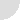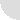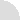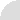LOGOLOG
a weblog of wordplay by Eric Harshbarger

## Letter Shifting and Summing

At least two of the wordplay related books I own (Language on Vacation by Dmitri A. Borgmann and Making the Alphabet Dance by Ross Eckler) discuss the idea of relating two words together by "letter shifting".

This involves shifting each letter of a word a set number of spaces "through the alphabet" and looking to see if the result is also a word. Eckler uses the following notation: fusion(6)layout to show that when each of the letters in "fusion" is shift 6 places forward in the alphabet, the resulting string of letters is the word "layout" (it is assumed the alphabet is cyclic, meaning that if a letter is shifted past the "Z", one wraps back around to "A" and continues the shift).

Eckler gives many examples of shifts of various lengths for various word lengths, as well as examples of chains of words which have been discovered [flap(13)sync(6)yeti]

The process of shifting the letters is a subtle way of thinking of the alphabet as an index wherein A=1, B=2, C=3,..., Z=26. The shift amounts to adding a constant to each numerical value (if that result exceeds 26, then subtract 26 to get it back into range). The new number is then back-translated to its corresponding letters and the result may or may not be a word.

With this in mind the topic can be extended in a direction I have not yet seen discussed (though, surely, someone has studied this earlier). If each letter in a word is associated with its indexing number, then, instead of shifting a constant number of spaces for each letter (like 6 in the "fusion" example above), one could shift each letter a different amount. But then, by how much for each letter? Well, each shift itself will be a number from 1 to 26 (a shift of 26 spaces will not really change the targeted letter), so each shift amount can have a letter of the alphabet associated with it. In affect, one is summing two words together to see if the result is a third word.

For example, consider the following four letter example:

```    WORD    NUMERICAL

EDIT     5, 4, 9,20  (because E=5, D=4,...)
+  NAIL    14, 1, 9,12
----    --,--,--,--
SERF    19, 5,18, 6  (20 + 12 == 32, -26 = 6)
```
Obviously, a computer can be helpful here (I wrote a quick program to analyze a long wordlist for all valid sums involving 4 to 20 letter words... that is how I got the examples for this page).

So, what is the longest length of two words that maybe summed to achieve a third word (obviously of equal length)? Well, I analzyed the web2.txt wordlist available from the National Puzzlers' League website...

-- Eric

[17 December 2005]Archive```Your Name:

Your comment (HTML tags will be removed):

Day of week today is:

```